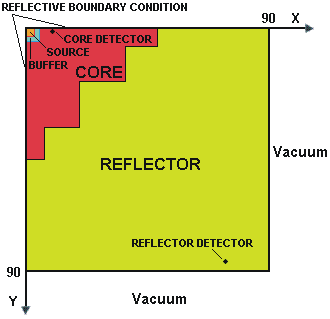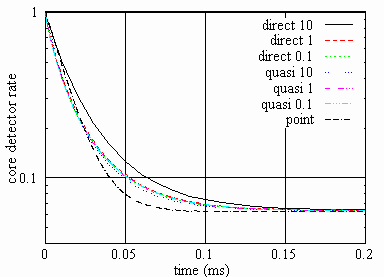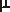# DEVELOPMENT OF KINETICS MODELS FOR TRANSIENT REACTOR TRANSIENT ANALYSES

Modeling of transient (i.e. time-dependent) reactor behavior is important for safety and neutronics experimental/diagnostics analyses.

In safety (accident) analyses time-dependent power distribution provided by kinetics models is employed for computing material temperature and density distributions in the reactor. On the other hand, these distributions are input data for computing the transient power. Thus a modern complex computer code for safety analyses (e.g. SIMMER) includes kinetics, thermal-hydraulics, structure and other models that interact during a transient simulation.

In neutronics experimental/diagnostics analyses, transients are modeled for certain reactor configurations that assumed to be known. Therefore, only neutron kinetics models can be employed.

In critical reactors for small deviations from the equilibrium, a point-kinetics model can be used that assumes constant neutron flux shape (i.e. spatial-energy-angular distribution of neutrons). That is why only transient flux amplitude should be computed. Thus the basic assumption of point-kinetics is:

f(x,t) = N(t)?(x)

i.e. the neutron flux (x represents position, energy and angle) is a product of amplitude (N) and a flux shape (?) that is time-independent.

For significant reactor perturbations (e.g. related to hypothetical severe accidents in safety analyses) the point-kinetics model is not accurate. This model is also inaccurate for transients in ADS caused by strong variations of the external neutron source or other perturbations. Another example - where a more refined kinetics treatment is needed - is a system with movable fuel (e.g. a molten salt reactor).

A more refined treatment may require assuming the flux shape and other distributions (e. g. delayed neutron precursor concentration shape) being time-dependent, e.g.

f(x,t) = N(t)?(x,t).

This approach may lead to a so-called quasistatic scheme. It assumes that the neutron flux shape is computed relative seldom while the neutron amplitude is computed much more often.

Since the equations for the flux shape are even more complex than those for the neutron flux, in case of very fast shape variations, one may prefer to solve directly (e.g. by employing a finite-difference scheme with respect to time) the "flux" equations. This gives rise to the so-called direct method that can serve (while employing very small time steps) as benchmark for other kinetics models. In the general case, however, this is the most time-consuming technique.

At FZK/IKET, the KIN3D (neutronics) and SIMMER (safety analysis) codes are under development. They employ all mentioned kinetics models and can be applied for analyses of innovative reactor systems suggested for transmutation.

 Examples:Figure 1. An experimental model with external neutron source, location of the detectors shownFigure 2. Core and reflector detector rate after source switch-off: computed with point-kinetics, quasistatic (employing shape steps of 0.1, 1, 10s) and direct options (similar time steps)